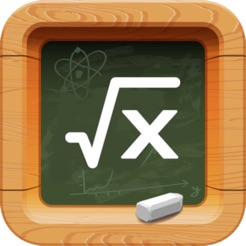## Screenshots

•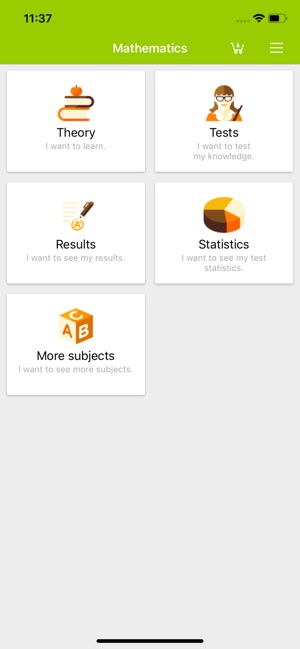•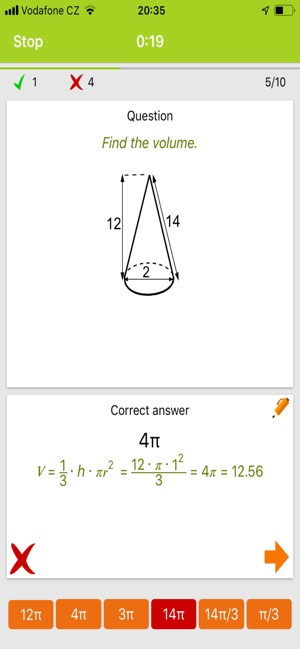•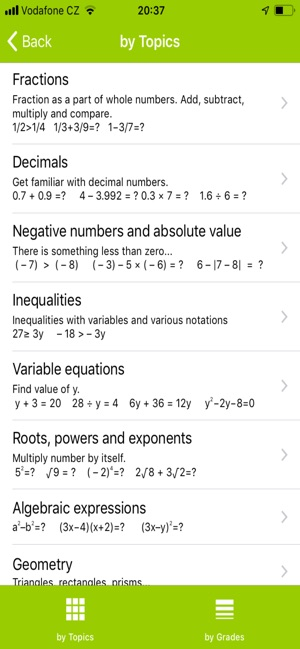•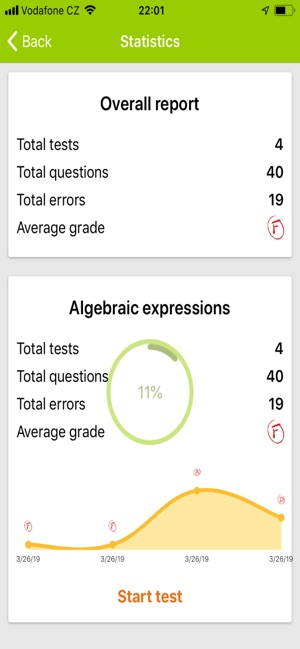••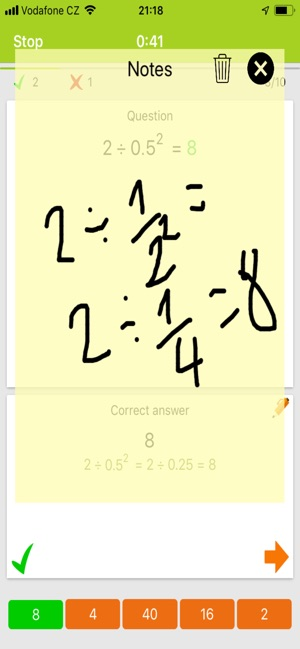•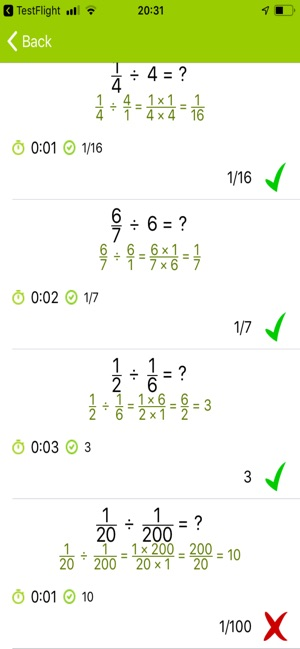•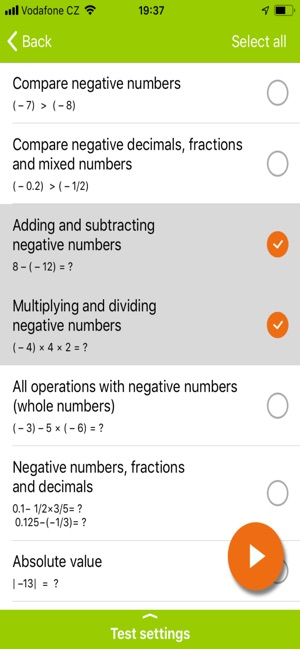## Description

Prepare for school exam by practicing with the most comprehensive math exercises.

Maths for children, students and adults!

Improve your math fluency through dozens of multiple-choice tests.
You get graded at the end of each test.

For 1st class/grade:
• adding and subtraction up to 100
• basic geometry 2D/3D figures and symmetry

For 2nd class/grade:
• multiplication and division
• ten based system and place value (expanded form, value of digits)
• metric units of measurements (time, length, weight, volume, area- meters, km, minutes, hours, liters, tons)
• US standard units of measures (cups-c,pints-pt,fl oz, yards-yd,inches-in,feet-ft,ounces, pounds-lb)
• converting am/pm time to 24 hour time

For 3rd class/grade
• subtraction, addition (even in columns with or without carrying), multiplication, division, divisibility, operations with brackets)
• rounding
• Roman numerals up to 1 000
• ten based system and place value (expanded form, value of digits)

For 4th class/grade:
• fraction practice (numerator and denominator, all operations, compare with whole numbers, mixed numbers, complex fractions, fractions in lowest term, adding and subtracting fractions, multiplying fractions, dividing fractions)
• rounding to whole tens, hundreds or thousands
• decimals (decimals and fractions, compare with fractions, multiplying and dividing decimals)

App also includes:
• divisibility (odd and even numbers, divisibility by 3, 4, 5, 6, 8, 9, 10)
• negative numbers and absolute value (compare negative numbers, add and subtract negative numbers, multiplying and dividing negative numbers, adding and subtracting absolute value)
• equations with variables (one-step equations, equations with negative numbers)
• inequalities and intervals (inequalities with all operations and negative numbers)
• geometry (number of sides, faces and vertices, area of squares, triangles, trapezoids and rhomboids, altitudes and medians, volume of prism, pyramid, cone, sphere, perimeter and area)
• square roots, powers and exponents (negative base, negative exponent, fractions as exponents, fractions as base, numerical and algebraic expressions with exponents, scientific notation)
• basic algebra
• sets, set operations, Venn diagrams and types of numbers
• percents %, ratios, cross-multiplication word problems, the rule of three, direct and indirect variation
• angles and their types (right, obtuse, acute; adjacent, vertical, linear pair, alternate, corresponding, consecutive), angles at the centre and circumference

This app is like having dozens of math worksheets and loads of exercises for 1st, 2nd, 3rd, 4th, 5th and 7th class directly in your device with results immediately available. It works offline so you learn to solve math and algebra problems whenever you want!

Key features overview:
• dozens of math categories and subcategories
• thousands of questions
• like in school, each test is evaluated and you are graded
• test history allows you to see where you made mistakes
• statistics showing your progress over time
------
IN-APP PURCHASE:
Get Eductify Math PRO version through an auto-renewing subscription.
Quarterly: \$3.49
Subscription terms:
• Payment will be charged to iTunes Account at confirmation of purchase
• Subscription automatically renews unless auto-renew is turned off at least 24-hours before the end of the current period
• Account will be charged for renewal within 24-hours prior to the end of the current period, and identify the cost of the renewal
• Subscriptions may be managed by the user and auto-renewal may be turned off by going to the user's Account Settings after purchase
• Any unused portion of a free trial period, if offered, will be forfeited when the user purchases a subscription to that publication, where applicable
------
Terms of use: https://www.holucent.com/eula/
Privacy policy: https://www.holucent.com/privacy-policy/

## What’s New

Version 1.1.1

- Added theory.
- New questions.
- Minor fixes and improvements.

## Information

Seller
Jiri Holubik
Size
60.4 MB
Category
Education
Compatibility

Requires iOS 11.2 or later. Compatible with iPhone, iPad, and iPod touch.

Languages

English, Czech, French, German, Japanese, Korean, Polish, Portuguese, Slovak, Spanish

Age Rating
Rated 4+
Copyright
© 2015 Holucent, Jiri Holubik
Price
Free
In-App Purchases
1. PRO version (lifetime) \$8.99
2. PRO version (3 months) \$3.49

## Supports

•